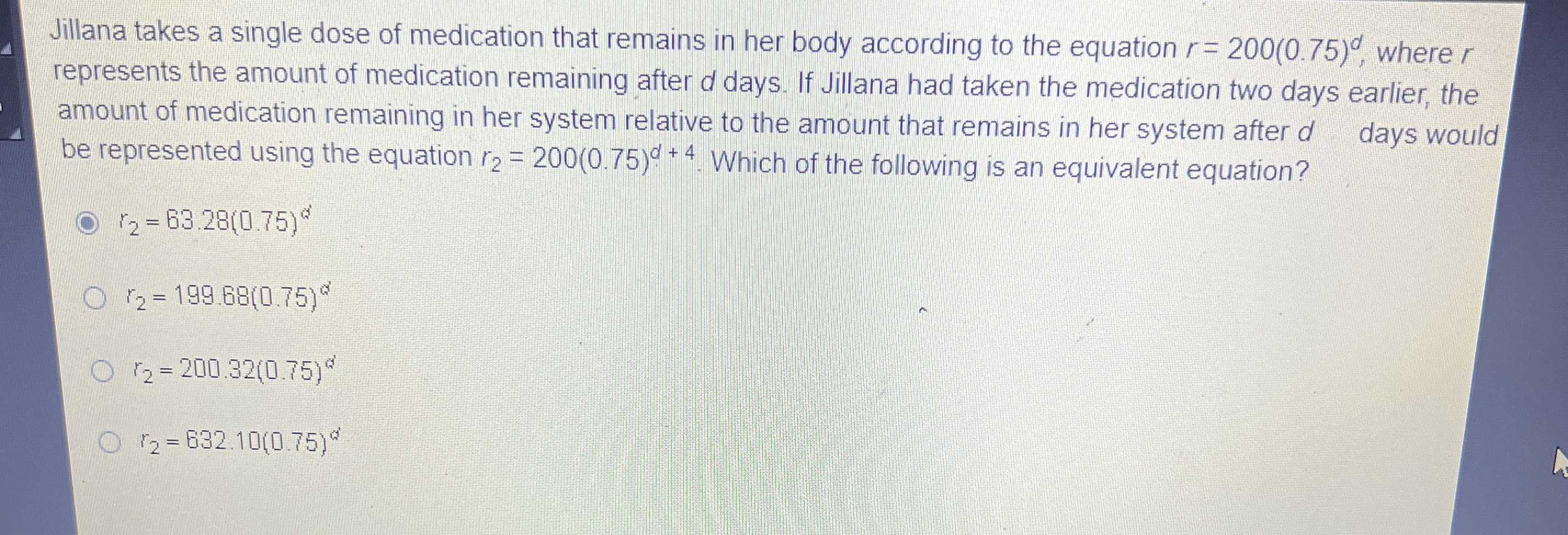### Still have math questions?

Algebra
QuestionJillana takes a single dose of medication that remains in her body according to the equation $$r = 200 ( 0.75 ) ^ { d }$$ , where $$r$$ represents the amount of medication remaining after d days. If Jillana had taken the medication two days earlier, the amount of medication remaining in her system relative to the amount that remains in her system after d days would be represented using the equation $$r _ { 2 } = 200 ( 0.75 ) ^ { d + 4 }$$ . Which of the following is an equivalent equation?

$$r _ { 2 } = 63.28 ( 0.75 ) ^ { d }$$

$$r _ { 2 } = 199.68 ( 0.75 ) ^ { d }$$

$$r _ { 2 } = 200.32 ( 0.75 ) ^ { d }$$

$$r _ { 2 } = 632.10 ( 0.75 ) ^ { d }$$